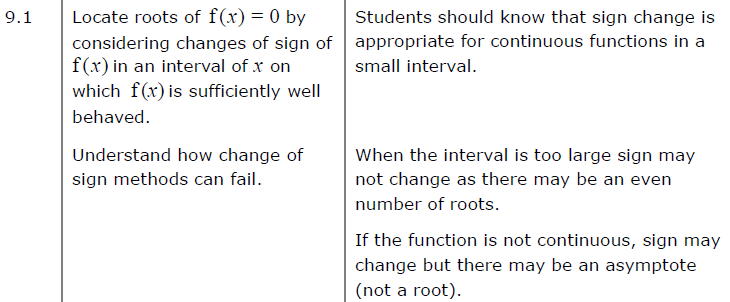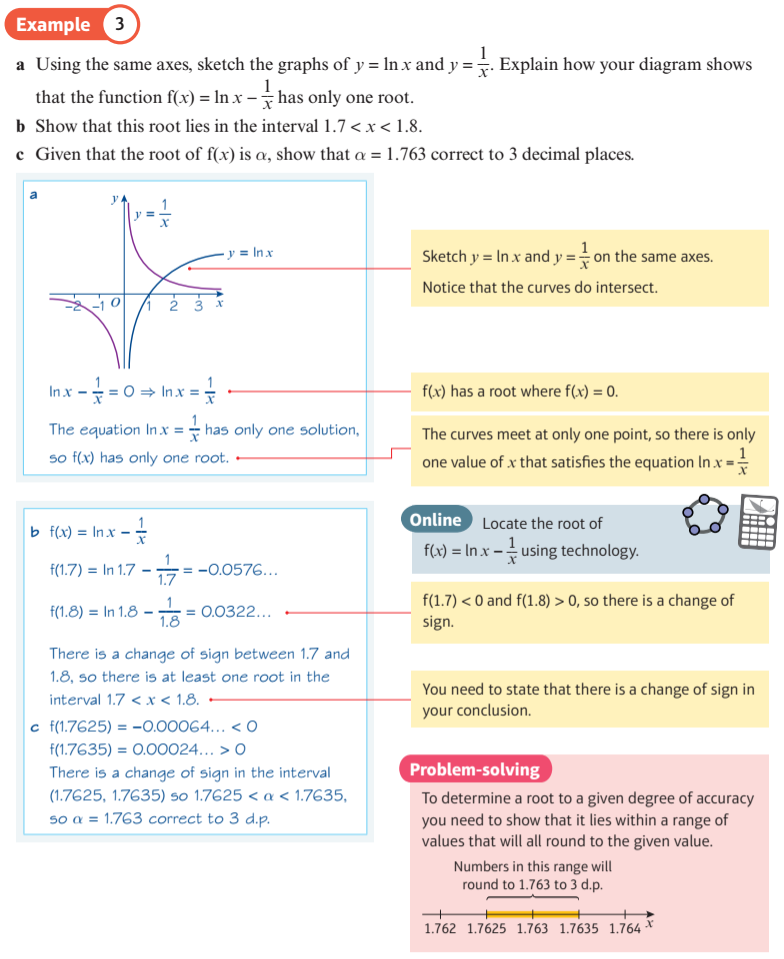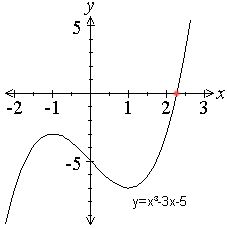Instant Maths Help:

# Edexcel A Level

## Locating Roots##### Zoom in on locating roots##### Change of Sign - Zooming In to Find RootsLet $$f(x) = {x^3} - 3x - 5$$.
To solve $${x^3} - 3x - 5 = 0$$ , we need to find where the $$f(x)$$ crosses the x-axis.

To do this we can display the graph $$f(x) = {x^3} - 3x - 5$$ using computer software or a graphic calculator, and zoom in on the interval where the curve crosses the x-axis.

In the display below, you can use the navigation button to zoom in on the crossing point. A crossing point between x = 2.25 and x = 2.30 means the solution is 2.3 to 1 decimal place. As you zoom in further, you can narrow the limits of the solution further, and therefore give the solution with an increasing number of decimal places accuracy. Can you give the solution to 4 decimal places, or maybe 5?

After you have solved the initial problem, you can change coefficient b to 3 (for multiple solutions), and then change all the coefficients to investigate other cubic curves.